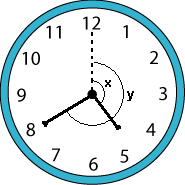#### You may also like### Consecutive Numbers

An investigation involving adding and subtracting sets of consecutive numbers. Lots to find out, lots to explore.### Calendar Capers

Choose any three by three square of dates on a calendar page...### Days and Dates

Investigate how you can work out what day of the week your birthday will be on next year, and the year after...

# Handy Angles

##### Age 11 to 14 Short Challenge Level:

Let $x$ denote the angle between the hour hand and the vertical, and $y$ the angle between the minute hand and the vertical.$\frac{360}{12}=30$ so we calculate that $x = \left( 4+\frac{2}{3} \right) \times 30 =140^\circ$ and $y = 8 \times 30 = 240^\circ$.

The angle between the hands is $y - x = 240^\circ - 140^\circ = 100^\circ$.
This problem is taken from the UKMT Mathematical Challenges.
You can find more short problems, arranged by curriculum topic, in our short problems collection.